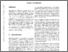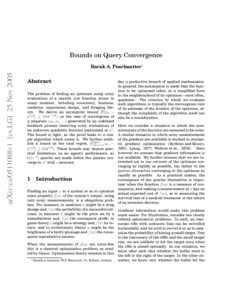# Bounds on Query Convergence

Pearlmutter, Barak A. (2005) Bounds on Query Convergence. Working Paper. arXiv.Previewmore...Add this article to your Mendeley library

## Abstract

The problem of finding an optimum using noisy evaluations of a smooth cost function arises in many contexts, including economics, business, medicine, experiment design, and foraging theory. We derive an asymptotic bound E[ (x_t - x*)^2 ] >= O(1/sqrt(t)) on the rate of convergence of a sequence (x_0, x_1, >...) generated by an unbiased feedback process observing noisy evaluations of an unknown quadratic function maximised at x*. The bound is tight, as the proof leads to a simple algorithm which meets it. We further establish a bound on the total regret, E[ sum_{i=1..t} (x_i - x*)^2 ] >= O(sqrt(t)) These bounds may impose practical limitations on an agent's performance, as O(eps^-4) queries are made before the queries converge to x* with eps accuracy.

Item Type: Monograph (Working Paper) Cite as: arXiv:cs/0511088 [cs.LG] Bounds; Query Convergence; Faculty of Science and Engineering > Computer Science 8163 arXiv:cs/0511088 [cs.LG] Barak Pearlmutter 13 Apr 2017 14:21 arXiv Science Foundation Ireland (SFI)Item control page